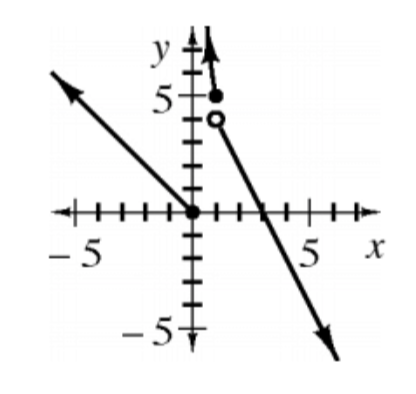### Home > APCALC > Chapter 1 > Lesson 1.2.1 > Problem1-21

1-21.

Given the functions below, compute the following values

1. $f ( x ) = \left\{ \begin{array} { c c c } { 4 - 3 x } & { \text { for } } & { x \leq 1 } \\ { x ^ { 2 } } & { \text { for } } & { x > 1 } \end{array} \right.$ Calculate $f(0)$, $f(1)$, and $f(3)$.

$x = 1$ can be called, informally, the Boundary Point of the piecewise function $f(x)$. This is because $x ≤ 1$ is the location where the left and the right pieces switch.

$f(0)$ and $f(1)$ are on the left side of the Boundary Point, $f(3)$ is on the right side of the Boundary Point.

$f\left(0\right) = 4 − 3\left(0\right) = 4$ LEFT PIECE
$f\left(1\right) = 4 − 3\left(1\right) = 1$ LEFT PIECE
$f\left(3\right) = \left(3\right)² = 9$ RIGHT PIECE

2. $g ( x ) = \left\{ \begin{array} { c c c } { \sqrt { x } } & { \text { for } } & { x < 3 } \\ { 3 - x } & { \text { for } } & { x \geq 3 } \end{array} \right.$Calculate $g(1)$, $g(3)$, and $g(9.4)$.

The Boundary Point is $x ≥ 3$. So $g(1)$ lies on the left side of the piecewise function while $g(3)$ and $g(9.4)$ lie on the right.

3. $h ( x ) = \left\{ \begin{array} { c c c } { - x } & { \text { for } } & { x \leq 0 } \\ { \frac { 5 } { x } } & { \text { for } } & { 0 < x \leq 1 } \\ { 6 - 2 x } & { \text { for } } & { x > 1 } \end{array} \right.$Calculate $h(–3)$, $h(0)$, $h(0.5)$, and $h(4)$.

This function has two boundary points and three pieces: a left piece, a middle piece and a right piece.

4. Sketch a graph of $y = h(x)$.